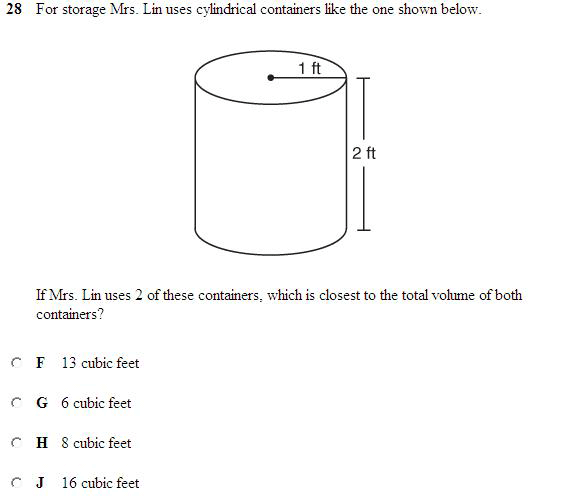SEARCH HOMEMath Central Quandaries & QueriesWhat is cubic feet and how do I use it in this question? (picture below)Hi there. Cubic feet is a measurement of volume (like gallons or litres). Volume is how we measure the amount of three dimensional space an object occupies.

So in the question you sent in, you need to answer how much space two of those oil drum-shaped things (we call that shape a "cylinder") take up.

Volume, because it is THREE dimensional, has three length components to it. For a box, we'd multiply the depth times the width times the height. The length of something can be measured in feet, so with all three length dimensions (width, depth and height) measured in feet we would be multiplying feet x feet x feet. That is feet^3 ("square feet" = feet^2 so "cubic feet" = feet^3). If all your length measurements are in feet, then you should expect that the volume you calculate will automatically be in cubic feet.

Now for a cylinder, there is a formula for the volume. It is this: 3.14 x radius x radius x depth. So you need to look at the diagram and figure out what is the radius and what is the depth. Then you can multiply using that formula and you will get the volume of one cylinder. If you double it, you will have the volume of two of those cylinders.

I suggest you ask your teacher for more help with the idea of "volume" because you will need to understand the concept well in order to understand questions asking you to calculate such things.

Hope this helps,
Stephen La Rocque.Math Central is supported by the University of Regina and The Pacific Institute for the Mathematical Sciences.